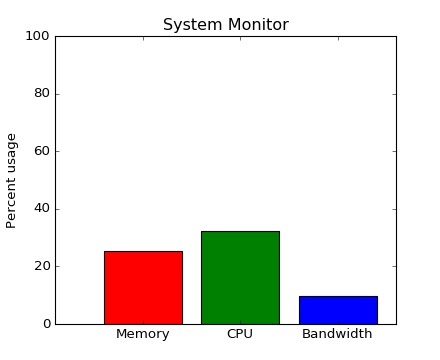### We're updating the default styles for Matplotlib 2.0

Learn what to expect in the new updates#### Previous topic

pylab_examples example code: symlog_demo.py

#### Next topic

pylab_examples example code: table_demo.py

# pylab_examples example code: system_monitor.py¶```import time
import matplotlib.pyplot as plt
import numpy as np

def get_memory():
"Simulate a function that returns system memory"
return 100*(0.5 + 0.5*np.sin(0.5*np.pi*time.time()))

def get_cpu():
"Simulate a function that returns cpu usage"
return 100*(0.5 + 0.5*np.sin(0.2*np.pi*(time.time() - 0.25)))

def get_net():
"Simulate a function that returns network bandwidth"
return 100*(0.5 + 0.5*np.sin(0.7*np.pi*(time.time() - 0.1)))

def get_stats():
return get_memory(), get_cpu(), get_net()

fig, ax = plt.subplots()
ind = np.arange(1, 4)

# show the figure, but do not block
plt.show(block=False)

pm, pc, pn = plt.bar(ind, get_stats())
centers = ind + 0.5*pm.get_width()
pm.set_facecolor('r')
pc.set_facecolor('g')
pn.set_facecolor('b')
ax.set_xlim([0.5, 4])
ax.set_xticks(centers)
ax.set_ylim([0, 100])
ax.set_xticklabels(['Memory', 'CPU', 'Bandwidth'])
ax.set_ylabel('Percent usage')
ax.set_title('System Monitor')

start = time.time()
for i in range(200):  # run for a little while
m, c, n = get_stats()

# update the animated artists
pm.set_height(m)
pc.set_height(c)
pn.set_height(n)

# ask the canvas to re-draw itself the next time it
# has a chance.
# For most of the GUI backends this adds an event to the queue
# of the GUI frameworks event loop.
fig.canvas.draw_idle()
try:
# make sure that the GUI framework has a chance to run its event loop
# and clear any GUI events.  This needs to be in a try/except block
# because the default implemenation of this method is to raise
# NotImplementedError
fig.canvas.flush_events()
except NotImplementedError:
pass

stop = time.time()
print("{fps:.1f} frames per second".format(fps=200 / (stop - start)))
```

Keywords: python, matplotlib, pylab, example, codex (see Search examples)